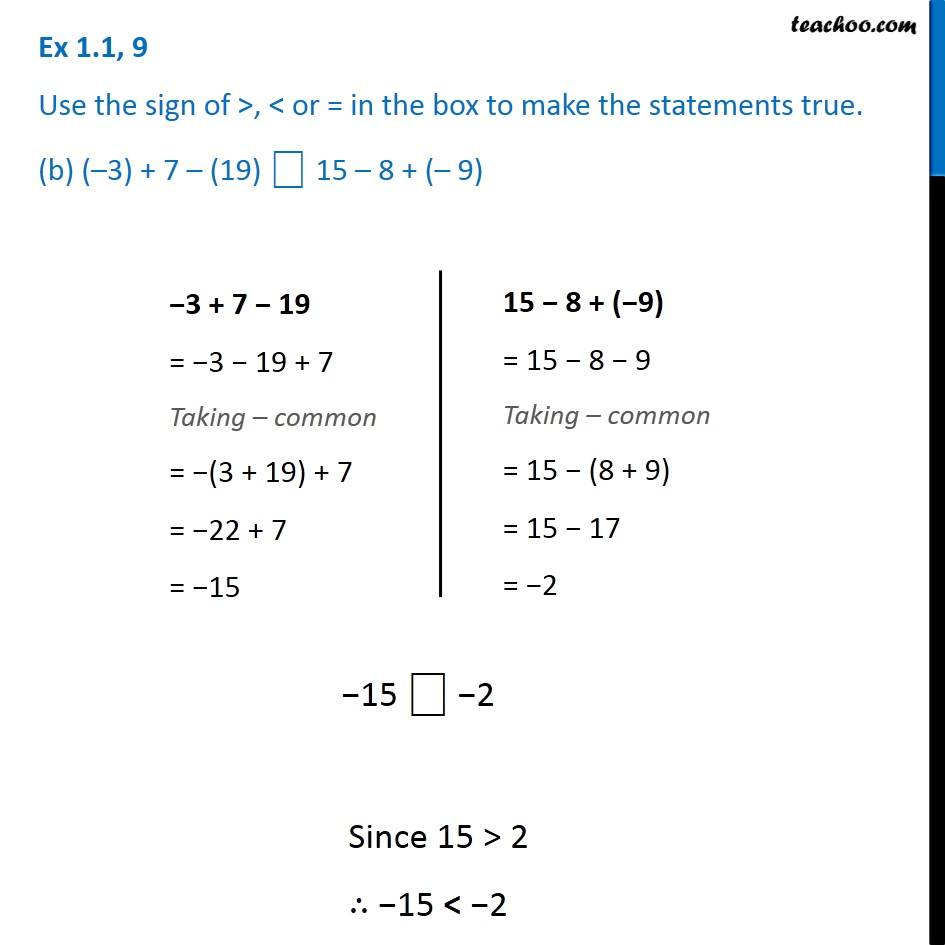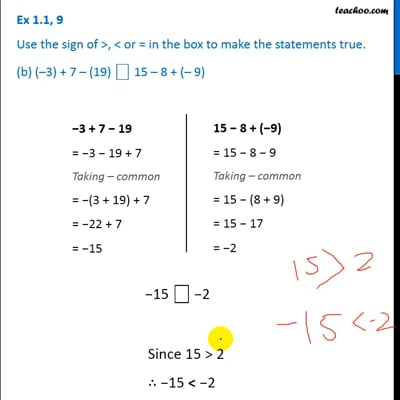Ex 1.1

Chapter 1 Class 7 Integers
Serial order wiseThis video is only available for Teachoo black users

Introducing your new favourite teacher - Teachoo Black, at only ₹83 per month

### Transcript

x 1.1, 9 Use the sign of >, < or = in the box to make the statements true. (b) (–3) + 7 – (19) ⎕ 15 – 8 + (– 9)−3 + 7 − 19 = −3 − 19 + 7 Taking – common = −(3 + 19) + 7 = −22 + 7 = −15 15 − 8 + (−9) = 15 − 8 − 9 Taking – common = 15 − (8 + 9) = 15 − 17 = −2 −15 ⎕ −2 Since 15 > 2 ∴ −15 < −2